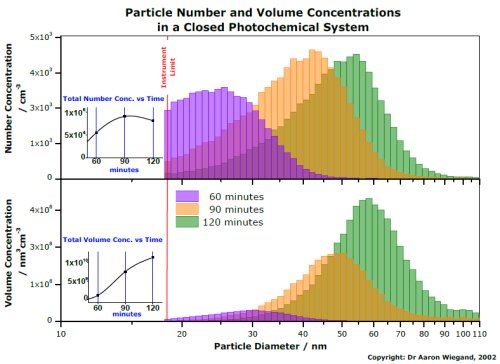Skip Navigation Links.

### Particle Analysis Study Shown in a 2 Panel Column/Bar Graph with Inset PlotsThis graph contains two types of plots. The colored "histogram-type" distribution plots represent the concentrations (number and volume) of particles as a function of diameter and the small line plots illustrate the TOTAL number and volume concentrations as a function of time. Total concentrations are calculated from the sum of the concentrations in the distribution plots, over all diameters (bins).

The right side shows how the concentration (number and volume) of particles changes in a photochemical system. The number concentration is important as it describes how many particles are in one cubic centimeter, and the volume concentration is important as it describes the actual amount (effectively the mass) of particles formed. The plot shows that although the total number (total area of each number distribution) of particles per cubic centimeter does not seem to increase a great deal over the second hour of this experiment (and in fact starts to decrease), the volume (mass) of particles does continue to increase. This is because chemicals condense onto the existing particles, rather than creating new particles, so the existing particles get bigger and heavier, thus increasing the particle SIZE and TOTAL volume as time goes by. The reason for the decrease in total aerosol number after 90 minutes is most likely due to coagulation: i.e. where two or more particles stick together (this reduces the total number, but has no effect on the total volume/mass).

The distribution plots (left side) are line-and-symbol plots, with no symbol displayed, but with "vertical drop lines" activated. The line-component of the distribution is of "step horizontal" type. The small line plots depicting total concentrations as a function of time are simple line plots, which are simply copied and pasted into the main graph.

Dr. Aaron Wiegand
Center for Instrumental & Develop. Chem.
"E" Block, Gardens Point Campus
Queensland University of Technology
GPO Box 2434, Brisbane 4001, AUSTRALIA